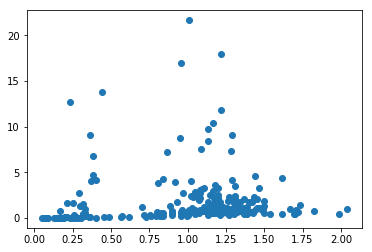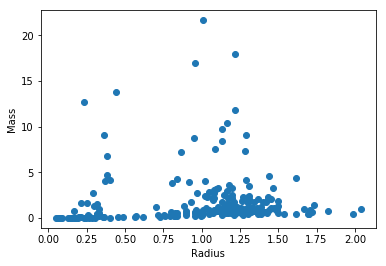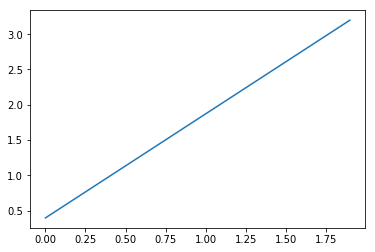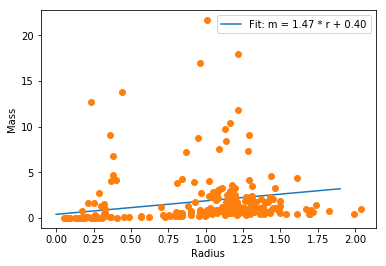(Skip this if you are working locally in Python or Jupyter notebook)

In :
from google.colab import files

print('User uploaded file "{name}" with length {length} bytes'.format(

Upload widget is only available when the cell has been executed in the current browser session. Please rerun this cell to enable.
Saving planets.txt to planets (3).txt
User uploaded file "planets.txt" with length 8898 bytes


Examine what this variable uploaded is

In :
uploaded.keys()

Out:
dict_keys(['planets.txt'])

Import numpy for math and number related functions, and matplotlib for plotting

In :
import numpy as np
from matplotlib import pyplot as plt


Open the file, loop through and build a dictionary with each planet's information

In :
planets = {}
f = open('planets.txt')
for line in f:
try:
name, mass, radius, period, nothing = line.split(',')
planets[name]={}
planets[name]['Mass'] = float(mass.strip('""'))
planets[name]['Period']= float(period.strip('""'))
except ValueError:
print(line)

"Kepler-33 b",,"0.16","5.66793",

"Kepler-33 c",,"0.29","13.17562",

"Kepler-33 d",,"0.48","21.77596",

"Kepler-33 e",,"0.36","31.7844",

"Kepler-33 f",,"0.4","41.02902",

"KIC 10905746 b",,"0.237","9.8844",

"KIC 6185331 b",,"0.72","49.76971",

"KOI-730 b",,"0.31","14.7903",

"KOI-730 c",,"0.23","9.8499",

"KOI-730 d",,"0.25","19.7216",

"KOI-730 e",,"0.18","7.3831",


In :
masses = []
for planet in planets:
try:
masses.append(planets[planet]['Mass'])
except:
continue

---------------------------------------------------------------------------
NameError                                 Traceback (most recent call last)
1 masses = []
----> 3 for planet in planets:
4   try:
5       masses.append(planets[planet]['Mass'])

NameError: name 'planets' is not defined
In :
planets[name]['Mass']

Out:
'1.077'
In :
plt.plot(radii,masses,'o')
plt.show()In :
plt.plot(radii, masses, 'o')
plt.ylabel('Mass')

Out:
Text(0, 0.5, 'Mass')In :
np.median(masses)

Out:
0.8574999999999999
In :
np.median(radii)

Out:
1.107
In :
np.polyfit?

In :
p = np.polyfit(radii,masses,1)

In :
print(p)

[0.39631182 1.47423546]

In :
fit_x = np.arange(0,2,0.1)
fit_y = p + p*fit_x
plt.plot(fit_x, fit_y)

Out:
[<matplotlib.lines.Line2D at 0x7fa19fc87048>]In :
plt.plot(fit_x,fit_y, label=str('Fit: m = %.2f * r + %.2f' % (p, p)))
plt.ylabel('Mass')
plt.legend(loc='best')

Out:
<matplotlib.legend.Legend at 0x7fa19ffe8c18>In :
import pandas as pd

In :
data = pd.read_csv('planets.txt')

In :
data = pd.

In :
data.describe()

Out:
Pl. Mass Pl. Radius Pl. Period Unnamed: 4
count 218.000000 229.000000 229.000000 0.0
mean 1.864573 0.953409 13.087551 NaN
std 3.061974 0.461101 35.579982 NaN
min 0.003000 0.051000 0.453285 NaN
25% 0.431500 0.490000 2.788491 NaN
50% 0.857500 1.090000 3.897130 NaN
75% 1.937000 1.281000 7.641590 NaN
max 21.660000 2.037000 289.862300 NaN
In :
data.columns

Out:
Index(['Planet Name', 'Pl. Mass', 'Pl. Radius', 'Pl. Period', 'Unnamed: 4'], dtype='object')
In :
plt.plot(data['Pl. Radius'],data['Pl. Mass'], 'o')

Out:
[<matplotlib.lines.Line2D at 0x7fa19fa34240>]